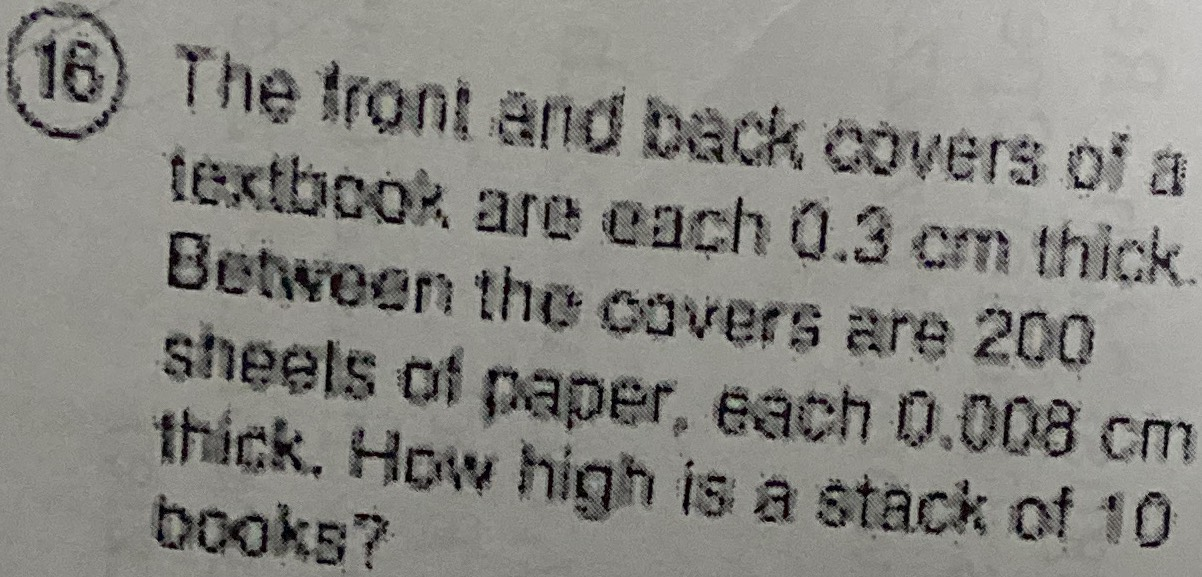### ¿Todavía tienes preguntas de matemáticas?

Pregunte a nuestros tutores expertos
Algebra
Pregunta(16) The lront and back covers of a textbook are each $$0.3 cm$$ thick. Betveen the ouvers are $$200$$ sheels of paper, each $$0.008 cm$$ thick. How high is a stack of $$10$$ books?